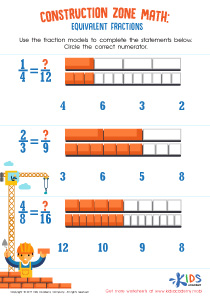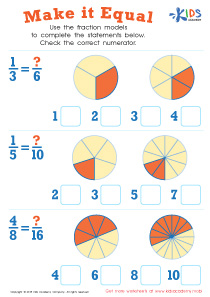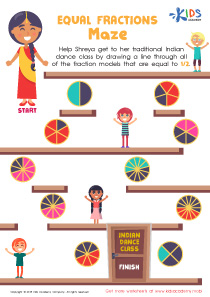# Math Lesson - Equivalent Fractions, Grade 3

• ### Activity 2 / Equivalent Fractions WorksheetFractions are easy to understand when kids are given simple pictures to represent each fraction! This equivalent fraction worksheet for 3rd grade uses colorful bricks in a construction zone to teach kids how to understand and compare fractions to find the numerator in each problem!

• ### Activity 3 / Make It Equal WorksheetUsing the fraction models in this worksheet, ask your kids to complete the statements written below the pictures. Then, check the correct numerator. In fraction, a numerator is the number that sits atop in the equation. There are three equations here that your kids will need to figure out. Each equation shows two pictures of a shape that has been divided in two different ways. Help them as they count the shaded parts of the shapes and check the correct numerators.

• ### Activity 4 / Equal Fractions Maze WorksheetShreya is the little girl your kids will be introduced to in this worksheet. She is Indian, and practices her Indian cultures and traditions, she needs to get to her Indian traditional dance class, and your little ones can help her by drawing a line through all of the fraction models that are equal to ½. Make sure that your kids start at the entrance marked ‘start’, and guide them through the maze paths with ½ until Shreya reaches the door to her Indian dance class.

?